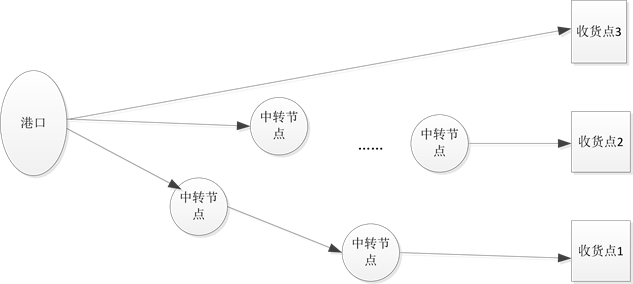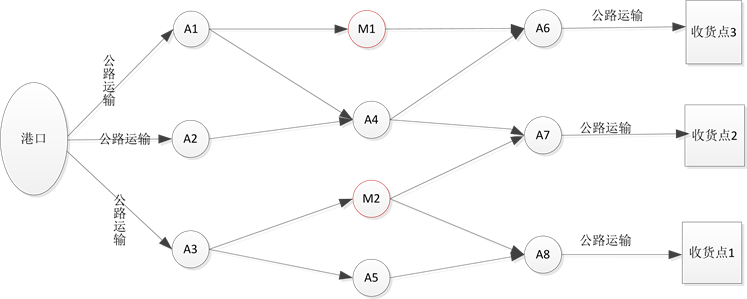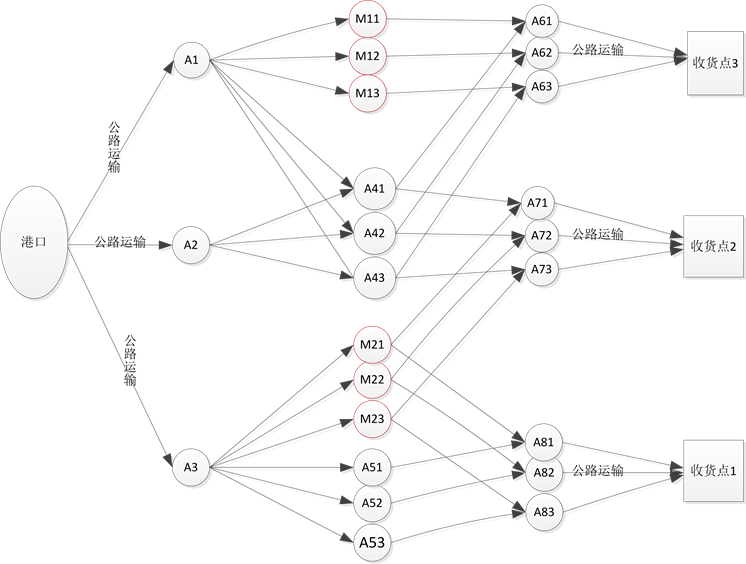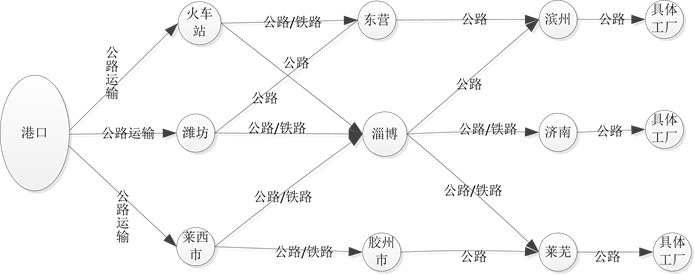﻿ 无车承运人的运输方式选择研究

# 无车承运人的运输方式选择研究Research on NTOCC’s Choice of Transportation Methods

Abstract: For NTOCC (non-truck operating common carrier), efficient organization for multiple modes of transport can reduce costs. From the perspective of NTOCC, this paper studies the choice of different modes of transport in multi-modal transport from four aspects: Transport cost, transit cost, time penalty cost and transportation safety cost. Taking into account capacity constraints, time constraints, the unit cost of different modes of transport and transport speed, we build the model with the lowest total cost, and solve with lingo10. The results show that the mode of transport options can effectively reduce costs.

1. 引言

2016年8月29日交通部发布了《关于推进改革试点 加快无车承运物流创新发展的意见》，明确规定无车承运存在的条件和法律地位，为促进物流货运行业的转型升级和提质增效创造了政策环境。试点的主要目的是期望无车承运人这种组织模式能够释放中国隐藏在地下的庞大的司机群体，实现组织的效率化和资源的无缝对接，真正解决物流行业的多年顽疾。通过去中间化，缩短交易的次数，实现各种等待时间的优化，实现真正意义的组织集约化的提升等。无车承运人指的是不拥有车辆而从事货物运输的个人或单位。对于真正的托运人来说，其是承运人；但是对于实际承运人而言，其又是托运人。无车承运一般不从事具体的运输业务，只从事运输组织、货物分拨、运输方式和运输线路的选择等  。无车承运人作为物流行业一种创新的发展模式，对于提升物流服务能力，推进物流供给侧结构性改革，促进物流业“降本增效”具有重要意义。

2. 问题描述与模型

2.1. 问题描述Figure 1. Port cargo transport routes

2.2. 模型的假设

1) 将无车承运的成本分为运输成本、中转成本、延迟惩罚成本、运输安全成本  四部分。

2) 两节点之间只能用一种运输方式运输。

3) 一个中转节点只能进行一种运输方式的转换。

4) 在中转节点进行转化时，既不发生破损，货运量也不会改变。

2.3. 参数说明

${C}_{ij}^{a}$ 从i点到j点采用a种运输方式的单位运输成本

${C}_{i}^{ab}$ 在i点由a种运输方式转为b种运输方式的单位中转成本

${L}_{ij}^{a}$ 从i点到j点采用a种运输方式的运输距离

${q}_{ij}^{a}$ 从i点到j点采用a种运输方式的运量

${q}_{i}^{ab}$ 在i点由a种运输方式转为b种运输方式运量

${Q}_{ij}^{a}$ 从i点到j点a运输方式的最大运力

${S}^{a}$ a种运输方式的运输安全成本

${C}_{t}$ 惩罚成本

${C}_{\text{0}}$ 单位惩罚成本

${t}_{ij}^{a}$ 从i点到j点采用a种运输方式的运输时间

${v}_{ij}^{a}$ 从i点到j点采用a种运输方式的运输速度

${t}_{i}^{ab}$ 在i点由a种运输方式转为b种运输方式的运输时间

$t$ 总运输时间

$T$ 客户要求时间

${Q}_{m}$ m个需求点

$A$ 共有A种运输方式

${x}_{ij}^{a}=\left\{\begin{array}{l}1\text{\hspace{0.17em}}\text{\hspace{0.17em}}\text{\hspace{0.17em}}\text{ }当i地到j地之间采取a种运输方式时\\ 0\text{\hspace{0.17em}}\text{\hspace{0.17em}}\text{ }\text{ }当i地到j地之间不采取a种运输方式时\end{array}$

${y}_{i}^{ab}=\left\{\begin{array}{l}1\text{\hspace{0.17em}}\text{\hspace{0.17em}}\text{\hspace{0.17em}}当i地由a种运输方式转为b种运输方式\\ 0\text{\hspace{0.17em}}\text{\hspace{0.17em}}\text{ }\text{ }当i地由a种运输方式不转为b种运输方式\end{array}$

2.4. 模型

$Z=\underset{i\in n}{\sum }\underset{j\in n}{\sum }\underset{a\in A}{\sum }{C}_{ij}^{a}{L}_{ij}^{a}{q}_{ij}^{a}{x}_{ij}^{a}+\underset{i\in n}{\sum }\underset{j\in n}{\sum }\underset{a\in A}{\sum }{C}_{i}^{ab}{q}_{i}^{ab}{y}_{i}^{ab}+{C}_{t}+\underset{i\in n}{\sum }\underset{j\in n}{\sum }\underset{a\in A}{\sum }{x}_{ij}^{a}{S}^{a}$ (1)

${C}_{t}=\left\{\begin{array}{l}0\text{\hspace{0.17em}}\text{\hspace{0.17em}}\text{\hspace{0.17em}}\text{\hspace{0.17em}}\text{\hspace{0.17em}}\text{\hspace{0.17em}}\text{\hspace{0.17em}}\text{\hspace{0.17em}}\text{\hspace{0.17em}}\text{\hspace{0.17em}}\text{\hspace{0.17em}}\text{\hspace{0.17em}}\text{\hspace{0.17em}}\text{ }\text{ }\text{ }t\le T\\ {C}_{0}\left(t-T\right)\text{\hspace{0.17em}}\text{\hspace{0.17em}}\text{\hspace{0.17em}}t\succ T\end{array}$ (2)

$t=\underset{i\in n}{\sum }\underset{j\in n}{\sum }\underset{a\in A}{\sum }{t}_{ij}^{a}+\underset{i\in n}{\sum }\underset{j\in n}{\sum }\underset{a\in A}{\sum }{t}_{i}^{ab}$ (3)

${t}_{ij}^{a}={L}_{ij}^{a}/{v}_{ij}^{a}$ (4)

$\underset{a\in A}{\sum }{x}_{ij}^{a}=1$ (5)

$\underset{a\in A}{\sum }\underset{b\in B}{\sum }{y}_{i}^{ab}=1$ (6)

${q}_{ij}^{a}\le {Q}_{ij}^{a}$ (7)

 (8)

 (9)

1) 是目标函数，由运输成本、中转成本、时间惩罚成本和运输安全成本构成。2) 是时间惩罚成本的计算方法。当总运输时间小于客户要求时间，时间惩罚成本为0。当总运输时间大于客户要求时间，时间惩罚成本为单位惩罚成本与延迟时间的乘积。3) 指总时间包括运输时间和中转时间。4) 运输时间等于运输距离除相应运输方式的运输速度。5) 指两个中转点之间只采用一种运输方式。6) 指一个中转节点只能进行一种运输方式的转换。7) 表示在i-j阶段a种运输方式的运量不超过其运力限制。8) 在中转节点转运时运入量等于运出量，保证了运输的连续性。9) 表示各种参数大于0。

3. 模型求解

3.1. 假设的运输网络图

3.2. 构建扩展的虚拟网络图Figure 2. Hypothesis of transport network diagramFigure 3. Expanded virtual network diagram

1) 为了简化模型的计算，在两城市之间有路径相连的城市，三种运输方式均可到达。

2) 为了方便计算，将城市进行扩展，每个城市扩展成3块，分别代表公路、水路、铁路运输。例如将A1扩展为A11/ A12/ A13。

3) 对于虚拟城市M1，假设A1-M1之间的运输时间、运输距离、运输费用为A1-A6之间的运输时间、运输距离、运输费用，而M1-A6之间的运输时间、运输距离、运输费用为0，并且M1-A6之间的运输能力无限大。

4) 对于没有弧连接的两城市，假设其运输时间、运输费用无限大，运输能力为0。

3.3. 求解

4. 算例分析

4.1. 成本数据收集

4.2. 运输数据采集

4.3. Lingo求解

Lingo运算的编程为：

!3 plants,3 warehouse and 3 customers

transshipment problem;

sets:

plant/p1,p2,p3/:produce;

warehouse/w1,w2,w3/;

customer/1,2,3/:require;

!the supply constraints;

@for(plant(i):[sup]

(@sum(warehouse(j):q11(i,j))+@sum(warehouse(j):q12(i,j)))=produce(i););

! the mid constraints;

@for(warehouse(j):[mid]

(@sum(plant(i):q11(i,j))+@sum(plant(i):q12(i,j)))=(@sum(customer(k):q21(j,k))+@sum(customer(k):q22(j,k)));

!the demand constraints;

@for (customer(k):[dem]

(@sum(warehouse(j):q21(j,k))+@sum(warehouse(j):q22(j,k)))=require(k));

EndFigure 4. Transport network diagramTable 1. Transit distances and capacity limits between transit nodesFigure 5. Final solution

5. 结语

 常连玉, 陈海燕. 无车承运人运力资源组织优化研究[J]. 公路交通科技, 2016, 33(10): 152-158.

 王巍, 张小东, 辛国栋. 基于多式联运的组合优化模型及求解方法[J]. 计算机工程与应用, 2009, 45(7): 212-214+219.

 谢海红, 刘瑛. 公铁联运配送方案优化[J]. 交通运输工程学报, 2011, 11(6): 89-93.

 乔欣宇. 基于成本分析的多式联运运输方式选择研究[D]. 西南交通大学, 2015.

 常连玉, 陈海燕. 无车承运人运力资源组织优化研究[J]. 公路交通科技, 2016, 33(10): 152-158.

 王巍, 张小东, 辛国栋. 基于多式联运的组合优化模型及求解方法[J]. 计算机工程与应用, 2009, 45(7): 212-214+219.

 谢海红, 刘瑛. 公铁联运配送方案优化[J]. 交通运输工程学报, 2011, 11(6): 89-93.

 乔欣宇. 基于成本分析的多式联运运输方式选择研究[D]. 西南交通大学, 2015.

Top